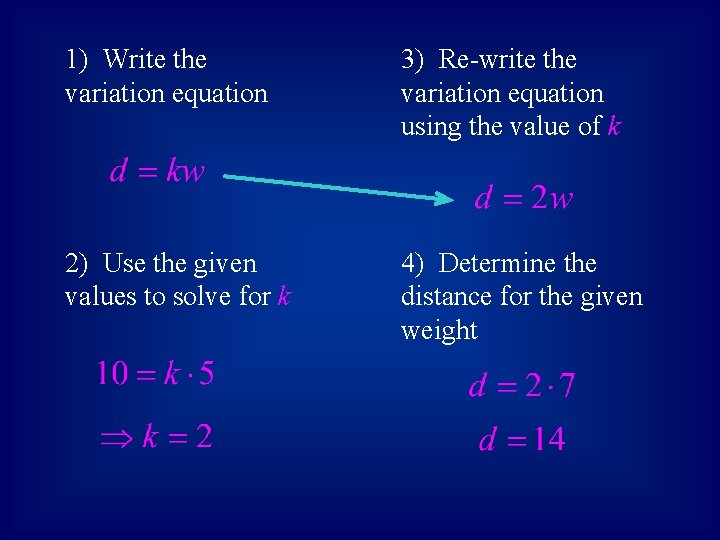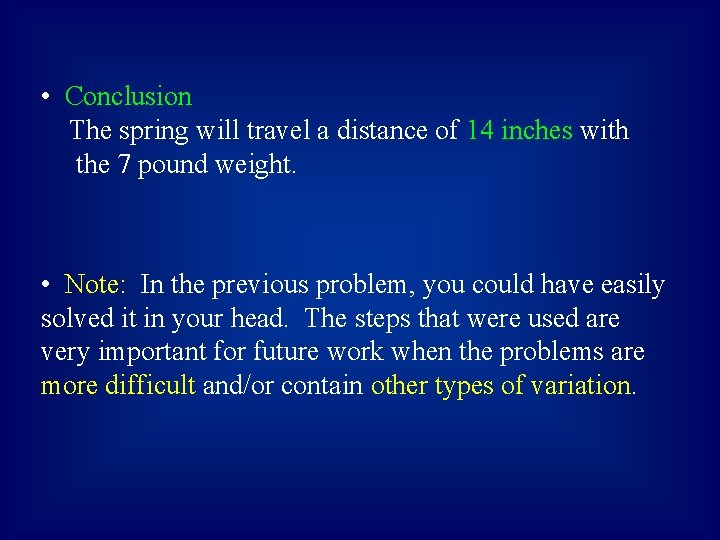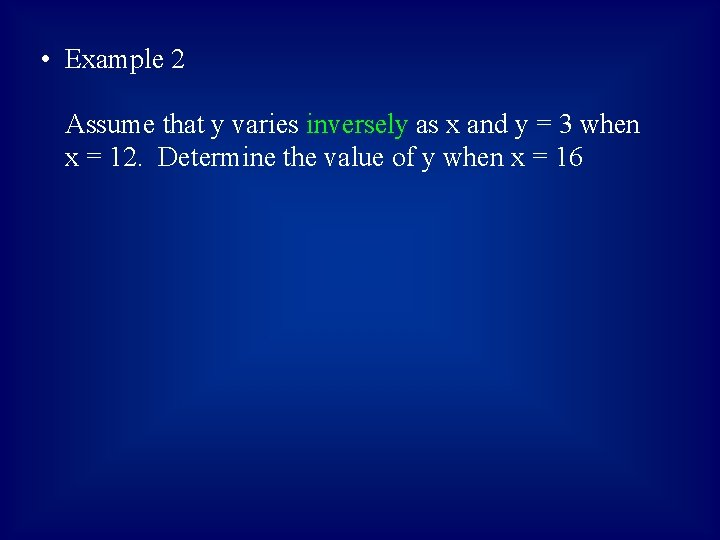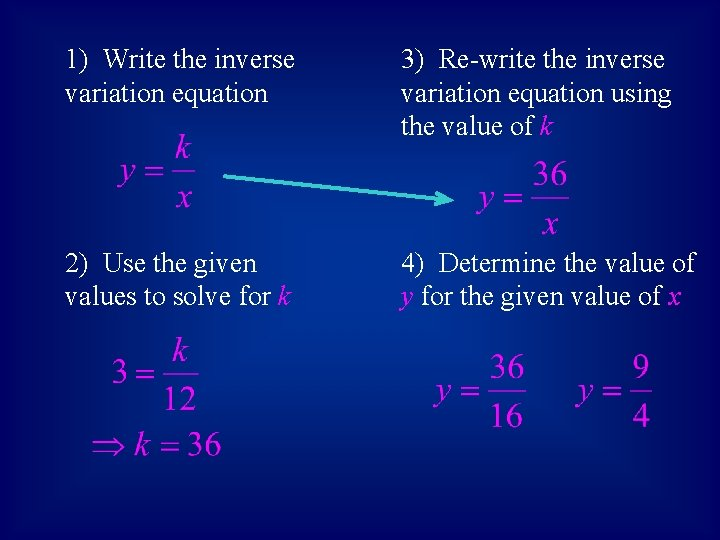Direct and Inverse Variation Direct Variation When y

• Slides: 8Direct and Inverse Variation • Direct Variation When y = k x for a nonzero constant k, we say that: 1. y varies directly as x, or 2. y is proportional to x The constant k is called the: 1. constant of variation, or 2. constant of proportionality• Example 1 When a weight is attached to a spring, the distance the spring stretches varies directly as the weight. If a weight of 5 pounds stretches the spring 10 inches, find the distance the spring will be stretched with a 7 pound weight. Let d = the distance the spring stretches w = the weight in pounds1) Write the variation equation 3) Re-write the variation equation using the value of k 2) Use the given values to solve for k 4) Determine the distance for the given weight• Conclusion The spring will travel a distance of 14 inches with the 7 pound weight. • Note: In the previous problem, you could have easily solved it in your head. The steps that were used are very important for future work when the problems are more difficult and/or contain other types of variation.• Inverse Variation When y = k/x for a nonzero constant k, we say that: 1. y varies inversely as x, or 2. y is inversely proportional to x The constant k is again called the: 1. constant of variation, or 2. constant of proportionality• Example 2 Assume that y varies inversely as x and y = 3 when x = 12. Determine the value of y when x = 161) Write the inverse variation equation 3) Re-write the inverse variation equation using the value of k 2) Use the given values to solve for k 4) Determine the value of y for the given value of x